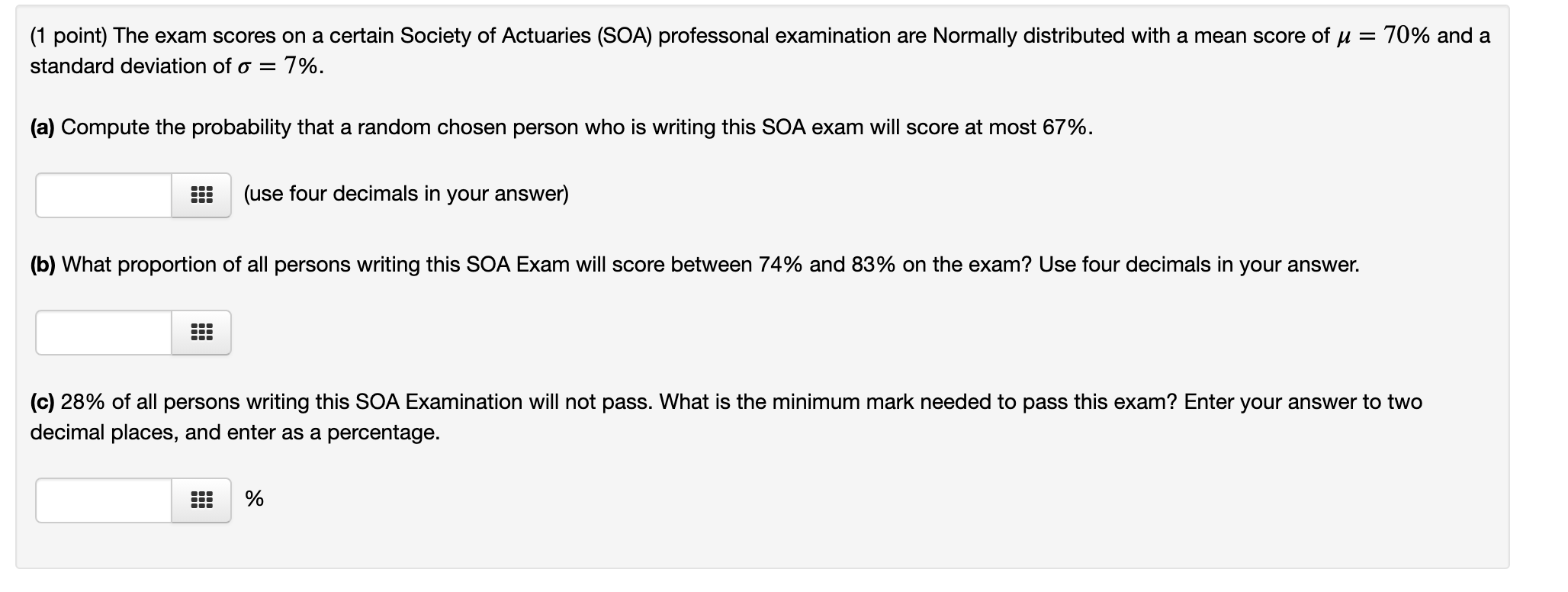# Question (1 point) The exam scores on a certain Society of Actuaries (SOA) professonal examination are Normally distributed with a mean score of j = 70% and a standard deviation of o = 7%. (a) Compute the probability that a random chosen person who is writing this SOA exam will score at most 67%. (use four decimals in your answer) (b) What proportion of all persons writing this SOA Exam will score between 74% and 83% on the exam? Use four decimals in your answer. (c) 28% of all persons writing this SOA Examination will not pass. What is the minimum mark needed to pass this exam? Enter your answer to two decimal places, and enter as a percentage. %5ZQX0P The Asker · Probability and StatisticsTranscribed Image Text: (1 point) The exam scores on a certain Society of Actuaries (SOA) professonal examination are Normally distributed with a mean score of j = 70% and a standard deviation of o = 7%. (a) Compute the probability that a random chosen person who is writing this SOA exam will score at most 67%. (use four decimals in your answer) (b) What proportion of all persons writing this SOA Exam will score between 74% and 83% on the exam? Use four decimals in your answer. (c) 28% of all persons writing this SOA Examination will not pass. What is the minimum mark needed to pass this exam? Enter your answer to two decimal places, and enter as a percentage. %
More
Transcribed Image Text: (1 point) The exam scores on a certain Society of Actuaries (SOA) professonal examination are Normally distributed with a mean score of j = 70% and a standard deviation of o = 7%. (a) Compute the probability that a random chosen person who is writing this SOA exam will score at most 67%. (use four decimals in your answer) (b) What proportion of all persons writing this SOA Exam will score between 74% and 83% on the exam? Use four decimals in your answer. (c) 28% of all persons writing this SOA Examination will not pass. What is the minimum mark needed to pass this exam? Enter your answer to two decimal places, and enter as a percentage. %